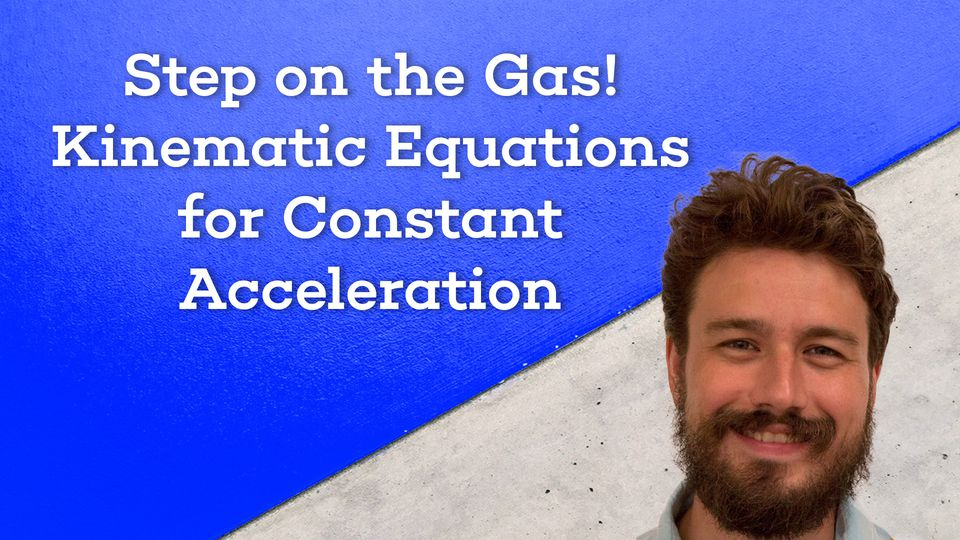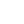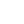Preview the Course
1h

# Constant Acceleration Formula and Kinematic Equations

by Robert Abramovic | Science FREE5 Lessons 1:00:22
1.1. Examples of Constant Acceleration 5:49
2.2. Velocity and Position Functions Derived From Constant Acceleration 9:11
3.3. An Object Moving for a Finite Duration of Time 14:21
4.4. Dimensional Movement: Time Dependent Equations 8:31
5.5. Dimensional Movement: A Time Independent Equation 22:30

1h

NGSS Standards

FREE

Course

The constant acceleration formula and the kinematic equations are all around us. Every time an object falls to the ground, its acceleration is 32 ft/s downward. Learning the kinematic equations for objects that accelerate at a constant rate is the first step in understanding projectile motion and the movement of dropped objects. In this course, you will learn how the vector-valued velocity and position functions are related to time. You will also learn about the special case of 1-dimensional movement and the distinction between time-dependent and time-independent kinematic equations.

Tags:

• Acceleration
• Functions
• Graphs
• Kinematics
• Math
• Physics
• Science
• STEM

## Instructor

Hi, I'm Robert. I hold a Ph.D. in Mathematics as well as a BA in Physics. I have been a private tutor for over 13 years. I enjoying sharing my knowledge of mathematics and physics with students, creating relatable examples, and making learning fun. My philosophy is not only teaching students but inspiring them to learn more about the material on their own.

See full profile